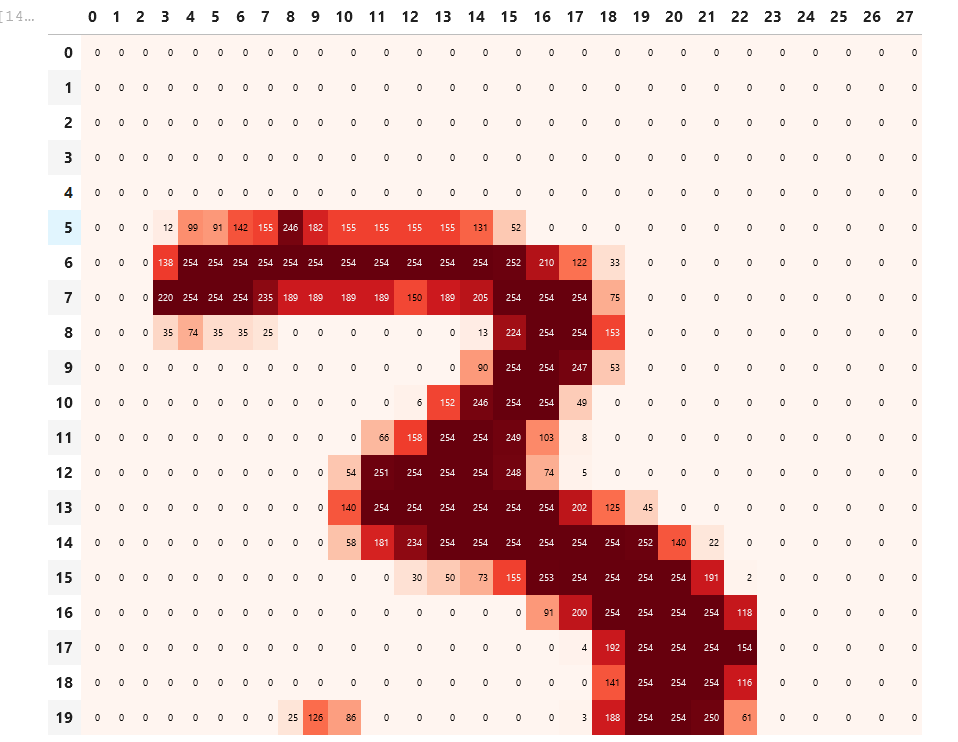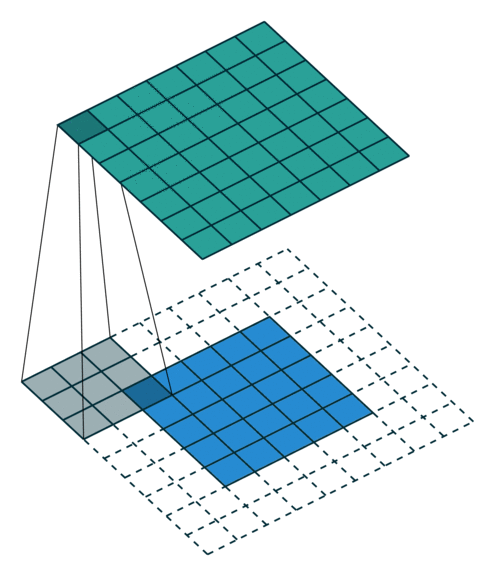The first section covered about filters. We used kernel which is a little matrix that is applied to the image which is used as a feature engineering step.

We have defined various version of filters, which can be used to extract features from the image like filters based on the top_edge:

top_edge = tensor([[-1, -1, -1], [0, 0, 0], [1, 1, 1]]).float()The above diagram shows how a three is actually represented actually in it’s pixel format.

To retain the orginal size, we usally use the following:

1. Padding - Add an extra layer to our existing image to add an extra layer of size. Such a padding layer can help in retaining our original image size to the same dimension.

2. Strides - Its step which the filter takes in each step.

To understand the concept of filters, and how different convolutions can be applied to notebook. I highly recommend to check out Ravi Mashru’s blogpost.

The general formula for output shape of a convolutional arthimetic is as shown:

``````= (input size + 2*pad - kernel size)// stride +1
``````

When applied to an image with full padding the result is as follows:``````= (input size + 2*pad - kernel size)// stride +1
= (5+ 2*2 - 3) + 1
= 7
``````

Understanding the input shape and output shape and why it’s comes that way is a challenging part of Pytorch.

``````top_edge = tensor([[-1,-1,-1],
[ 0, 0, 0],
[ 1, 1, 1]]).float()
left_edge = tensor([[-1,1,0],
[-1,1,0],
[-1,1,0]]).float()
diag1_edge = tensor([[ 0,-1, 1],
[-1, 1, 0],
[ 1, 0, 0]]).float()
diag2_edge = tensor([[ 1,-1, 0],
[ 0, 1,-1],
[ 0, 0, 1]]).float()

edge_kernels = torch.stack([left_edge, top_edge, diag1_edge, diag2_edge])

mnist = DataBlock((ImageBlock(cls=PILImageBW), CategoryBlock),
get_items=get_image_files,
splitter=GrandparentSplitter(),
get_y=parent_label)

xb,yb = first(dls.valid)
xb.shape
``````

torch.Size([64, 1, 28, 28])

``````edge_kernels.shape,edge_kernels.unsqueeze(1).shape
``````

(torch.Size([4, 3, 3]), torch.Size([4, 1, 3, 3]))

Then we are looking at the applying convolutions in pytorch. The edges of each shape of `28*28 size images` when applied to a filter are returning 64 batches of 4 filters and height and weight is `26*26` as it loses 2 pixels.

If you look at the reference link, you will realize how actually the matrix multiplication in our convolution is actually happening.

Also another interesting update for this week is the plan to create a movie recommender. Ravi Mashru and I had a call to kick off the project, which we are planning to complete by investing 2-4 hours per week. More about it can be found here.

• https://medium.com/impactai/cnns-from-different-viewpoints-fab7f52d159c